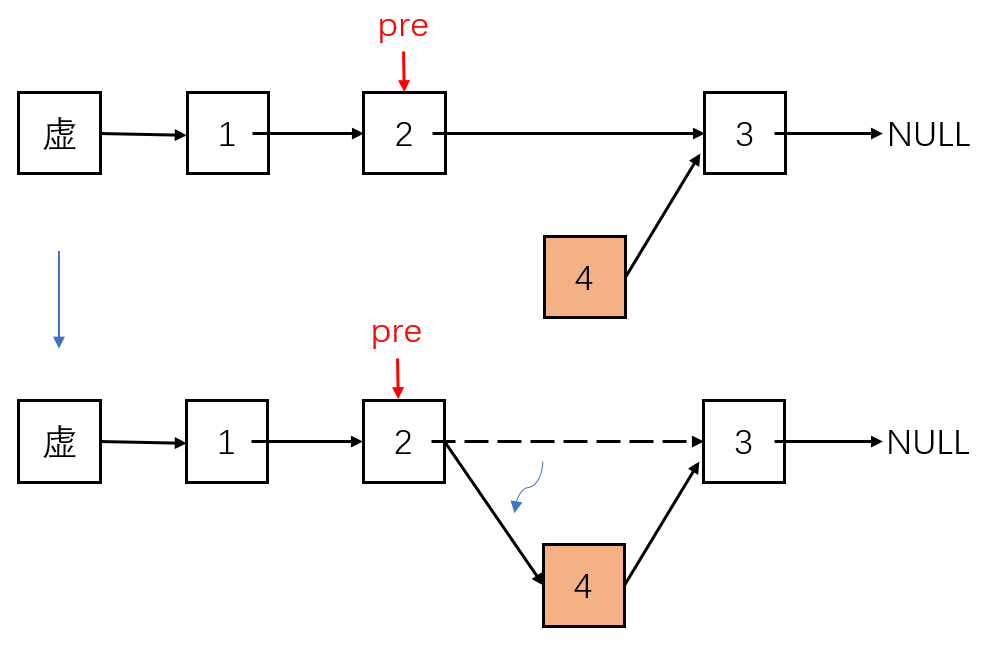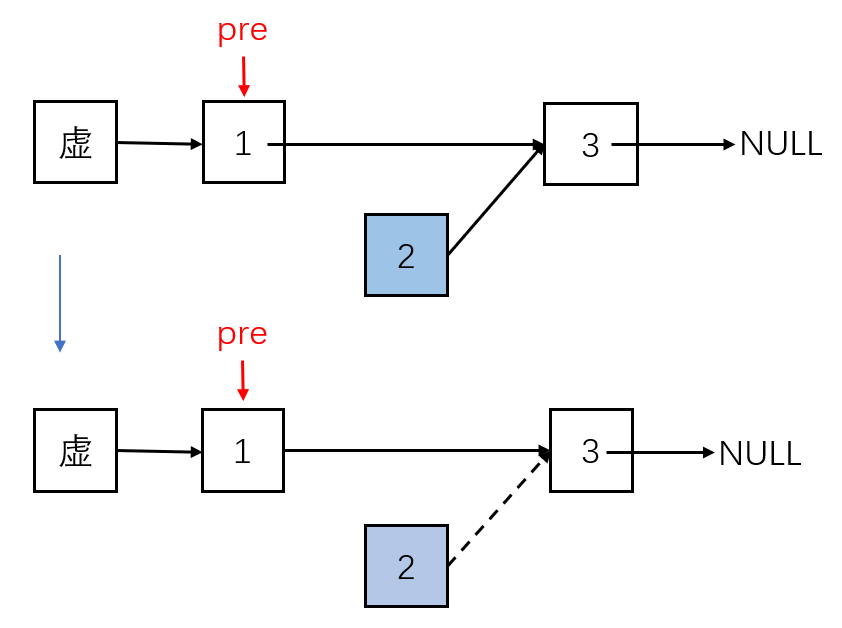Github LiYangSir
Home https://quguai.cn
Language Java

1、什么是链表

class Node{
E e;
Node next;
}1.1、同数组的区别

• 优点：具备随机访问能力，直接根据地址寻址一步完成
• 缺点：不具备真正的动态性，底层依然依靠固定容量的数组

• 优点：具有真正的动态性，完全根据用户的数据建立节点
• 缺点：缺少了随机访问能力，不适合应对查询元素频繁的场景

1.2、节点的实现

private class Node{  //
public Node(E e, Node node) {
this.e = e;
this.next = node;
}

public Node(E e) {
this(e, null);
}

public Node() {
this(null, null);
}

@Override
public String toString() {
return e.toString();
}

E e;
Node next;
}
}

2、链表的方法实现

2.1、增加元素

1. 找到要插入位置的前一个节点pre
2. 新增节点，并指向pre节点的下一个节点
3. 将pre节点指向新的节点public void add(int index, E e) {
if (index < 0 || index > size)  //越界判断
throw new IllegalArgumentException("add is error, index need index < 0 || index > size ");
for (int i = 0; i < index; ++i)   //找到插入位置的前一个节点
pre = pre.next;
pre.next = new table(e, pre.next);
size++;
}

2.2、删除元素

1. 找到索引位置的前一个节点pre
2. pre节点指向pre节点的下一节点的下一个节点
3. 断开待删除节点与其下一个节点的连接，删除的节点会被内存管理机制自动销毁public E remove(int index){
if (index < 0 || index > size)
throw new IllegalArgumentException("set is error, index need index < 0 || index > size ");
for (int i = 0; i < index; ++i)  //找到待删除节点的前一个节点
pre = pre.next;
size--;
table retNode = pre.next; //保存待删除节点，后面还要返回
pre.next = retNode.next;
retNode.next = null;  //断开连接
return retNode.e;
}

public void remove(int index){
if (index < 0 || index > size)
throw new IllegalArgumentException("set is error, index need index < 0 || index > size ");
for (int i = 0; i < index; ++i)
pre = pre.next;
size--;
pre.next = pre.next.next;  //直接删除
}

2.3、改变元素

public void set(int index, E e) {
if (index < 0 || index > size)
throw new IllegalArgumentException("set is error, index need index < 0 || index > size ");
for (int i = 0; i < index; ++i)  //找到元素
pre = pre.next;
pre.e = e;   //改变元素
}

2.4、查询元素

1. find(E e) 查询元素e所在位置
2. contains(E e) 查询是否包含元素，同find代码逻辑相同
3. get(int index) 查询index位置上的元素
/**    是否包含元素    **/
public boolean contains(E e) {
while (currentTable != null) {
if (currentTable.e == e)
return true;
currentTable = currentTable.next;
}
return false;
}
/**    查找元素所在位置    **/
public int find(E e) {
int index = 0;
while (currentTable != null) {
if (currentTable.e == e)
return index;
index++;
currentTable = currentTable.next;
}
return -1;
}
/**      查询index位置的元素    **/
public E get(int index) {
if (index < 0 || index > size)
throw new IllegalArgumentException("get is error, index need index < 0 || index > size ");
for (int i = 0; i < index; ++i)
pre = pre.next;
return pre.e;
}

最后